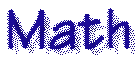Algebra Help Beat the Calculator - Math tips and tricks Division Tips Dave's Math Tables Dr. Math Fractions Geometry-Formulas Magic Squares Math Math Exercises Math Forum Math Homework Help MathMagic Measurement Resources Mega Math Multiplication Table Multiplying Tips S.O.S. Math - best site for High School Math The Abacus Extremekids.com® Copyright © 2009 All Rights Reserved.